Translating from Word Statements

 Problem Set 1 Problem Set 2 Problem Set 3Select a problem set using the buttons above, then use your mouse or tab key to select a question. Fill in the blank with the correct answer for each problem. When you have answered all of the questions, ask Charlie how you did.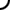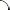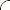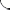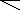Translate each word statement to a mathematical expression.   The sum of two and six. The sum of x and five. The difference of seven and three. The difference of four and y. The sum of one, x, and eight is twenty four. The difference of z and five is twelve.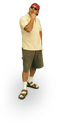Translate each word statement to mathematical expressions.   The sum of eleven and eight. The sum of one and x. The difference of seven and two. The difference of x and five. The sum of seven, four, and y is thirteen. The difference of twelve and z is fourteen.Translate each word statement to mathematical expressions.   The sum of seven and eight. The sum of y and nine. The difference of three and one. The difference of six and z. The sum of three, w, and four is seventeen. The difference of x and two is ten.©  2009  LarryPerezWeb Design:  Patrick Quigley